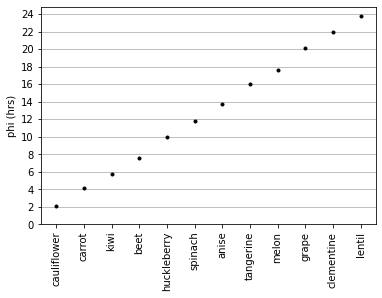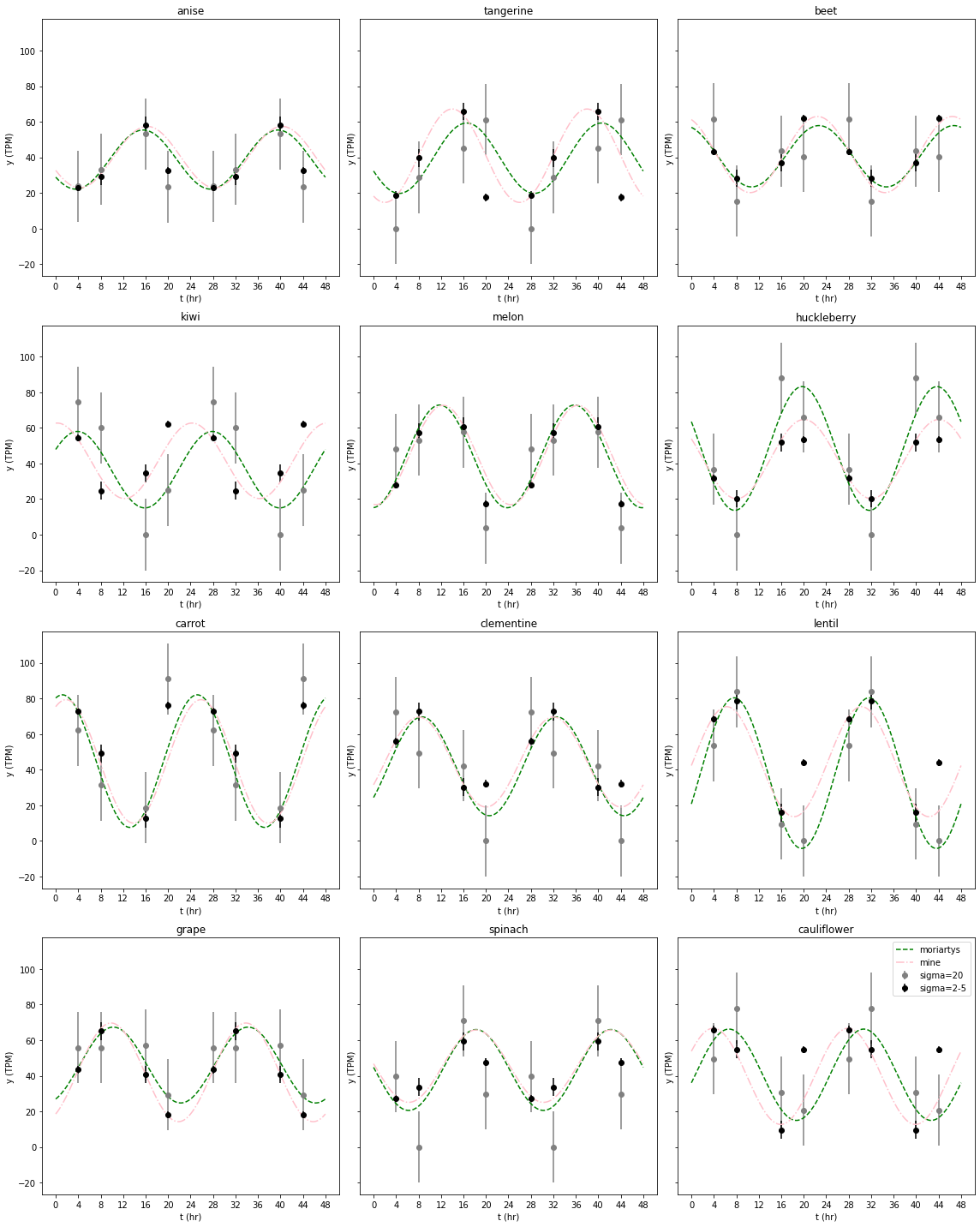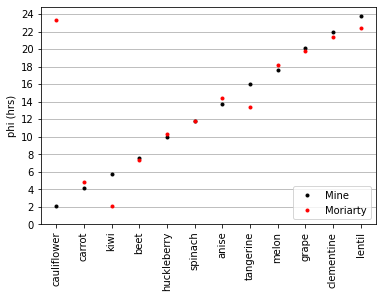Problem Set 08¶

In :
import numpy as np
import pandas as pd
from matplotlib import pyplot as plt
import scipy.optimize as optimize
import scipy.stats as stats
import math
import re   #for moriorty.py

1. Solve by maximum likelihood¶

Let's first inspect the data.

In :
!cat "w08-data.tbl"
4hr    4hr    8hr    8hr   16hr   16hr   24hr   24hr
gene           +-20    +-2   +-20    +-5    +-5   +-20    +-2   +-20
------------ ------ ------ ------ ------ ------ ------ ------ ------
anise         23.76  23.19  33.33  29.36  58.09  53.23  32.63  23.48
kiwi          74.46  54.53  59.97  24.63  34.45   0.00  62.12  25.04
carrot        62.08  72.83  31.45  49.08  12.61  18.49  76.04  91.07
grape         55.74  43.46  55.83  65.21  40.75  57.33  18.13  29.24
tangerine      0.00  18.83  28.75  39.69  65.80  45.29  17.48  61.24
melon         48.00  27.93  53.07  57.49  60.84  57.65  17.38   3.83
clementine    72.22  56.16  49.29  72.70  30.23  42.15  32.11   0.00
spinach       39.62  27.26   0.00  33.72  59.37  70.99  47.72  29.73
beet          61.48  43.18  15.43  28.25  37.14  43.53  61.96  40.37
huckleberry   36.77  31.89   0.00  20.23  51.81  87.98  53.55  66.11
lentil        53.58  68.50  83.86  78.84  15.83   9.53  43.90   0.00
cauliflower   49.54  65.97  77.94  54.80   9.68  30.73  54.75  20.60

So we have a file with expression levels for particular genes, and their time and uncertaintt on the first 2 lines. Let's read this into a dataframe and record the times and sigmas in an array as well.

In :
t = np.array([4,4,8,8,16,16,24,24])
sigmas = np.array([20,2,20,5,5,20,2,20])

#general parameters
omega = 1/24
pi = math.pi
In :
Out:
1 2 3 4 5 6 7 8
anise 23.76 23.19 33.33 29.36 58.09 53.23 32.63 23.48
kiwi 74.46 54.53 59.97 24.63 34.45 0.00 62.12 25.04
carrot 62.08 72.83 31.45 49.08 12.61 18.49 76.04 91.07
grape 55.74 43.46 55.83 65.21 40.75 57.33 18.13 29.24
tangerine 0.00 18.83 28.75 39.69 65.80 45.29 17.48 61.24

We want to see if the model of circadian rhythm can actually explain the data. For that, aim to maximise the likelihood, $P(D|\theta)$.

$P(D|\theta) = P(D_1|\theta_1)+P(D_2|\theta_2)+...+P(D_n|\theta_n) = \prod_{i=1}^{n} P(D_i|\theta_i)$

Since these will be very small numbers, we will work with the log.

$log P(D|\theta) = log \sum_{i=1}^{n} P(D_i|\theta_i)$

We model the cyclic expression with $y_t = b + A sin (2\pi\omega(t+\phi))$.

We can then calculate the likelihood by assuming the error is Gaussian distributed, and calculating the probability density function over the difference in data point and calculated expression, given the standard deviation of that point (which we know).

$P(D_i|\theta_i) = \frac{1}{\sqrt{2 \pi \sigma_i^2}}exp[\frac{y_{i(obs)}-y_{i(calc)}}{2 \pi \sigma_i^2}]$

Thus

$log P(D|\theta) = log \prod_{i=1}^{n} \frac{1}{\sqrt{2 \pi \sigma_i^2}}exp[\frac{y_{i(obs)}-y_{i(calc)}}{2 \pi \sigma_i^2}]$

where $y_{i(obs)}$ is the observed datapoint and $y_{i(calc)}$ is the calculated datapoint with $y_{i(calc)} = b + A sin (2\pi\omega(t_i+\phi))$ .

We can then optimise this likelihood, and retrieve the optimal parameters b, a and phi.

In :
def nll(p, data_row):
'''
Calculated the negative log likelihood given the data and parameters b,a,and phi in vector p.
Negative ll is given for usage of function in scipy.optimize.minimize.

Input:
p (vector): vector with 3 values: b,a,phi.
data_row (np array): array with 8 data points for a particular gene.

Returns:
-np.sum(ll_row) (float): negative log likelihood.
'''
curr_b, curr_a, curr_phi = p
calc_x_row = curr_b + curr_a * np.sin(2*pi*omega*(t+curr_phi))
res_row = data_row - calc_x_row
ll_row  = stats.norm.logpdf(res_row, 0, sigmas)
return -np.sum(ll_row)
In :
def optimise(data_row):
'''
Function for retrieving parameters b,a and phi such that the likelihood is maximised compared to the data.
Uses scipy.optimize.minimize. 0

Input:
data_row (np array): array with 8 data points for a particular gene.

Returns:
b_fit (float): b such that likelihood is optimised.
a_fit (float): a such that likelihood is optimised.
phi_fit (float): phi such that likelihood is optimised.
ll_fit (float): likelihood of the data in data_row given the optimal parameters b_fit,a_fit and phi_fit.

'''
p0 = np.array([10,10,10])                              #initial guess does not really matter.
result = optimize.minimize(nll, p0, (data_row))
if result.success != True:
print("Maximum likelihood fit failed")
else:
b_fit = result.x
a_fit = result.x
phi_fit = result.x

#phi is the offset, and can either be positive or negative (due to the nature of a sinus function). This ensures all phi's are returned positive, so it's easier to compare them all.
if phi_fit < 0:
phi_fit = 24 + phi_fit

ll_fit = -nll(result.x, data_row)
return b_fit,a_fit,phi_fit,ll_fit
In :
Probs_dict = {}

for row in df.iterrows():
gene = str(row)
data_row = np.array(row[1:8])
b_fit,a_fit,phi_fit,ll_fit = optimise(data_row)

Probs_dict[gene] = b_fit,a_fit,phi_fit,ll_fit

df_probs = pd.DataFrame.from_dict(Probs_dict,orient='index',columns=["b","a","phi","ll"])
df_probs
Out:
b a phi ll
anise 40.172735 17.244439 13.715488 -24.118830
kiwi 41.515034 21.208754 5.739874 -30.143606
carrot 44.654608 34.676007 4.158166 -25.657116
grape 41.954840 27.715396 20.159634 -24.837582
tangerine 40.927141 26.241822 15.993743 -27.513061
melon 44.800178 27.890750 17.599526 -24.745585
clementine 44.288956 25.270135 21.972219 -26.465672
spinach 45.430155 20.334596 11.747027 -26.816629
beet 41.577986 21.381025 7.543745 -25.522615
huckleberry 42.485342 22.244470 9.946389 -26.240205
lentil 44.489946 30.892248 23.730146 -28.478018
cauliflower 39.703344 27.012666 2.099127 -27.353093
In :
df_probs.sort_values(by="phi",axis=0)
Out:
b a phi ll
cauliflower 39.703344 27.012666 2.099127 -27.353093
carrot 44.654608 34.676007 4.158166 -25.657116
kiwi 41.515034 21.208754 5.739874 -30.143606
beet 41.577986 21.381025 7.543745 -25.522615
huckleberry 42.485342 22.244470 9.946389 -26.240205
spinach 45.430155 20.334596 11.747027 -26.816629
anise 40.172735 17.244439 13.715488 -24.118830
tangerine 40.927141 26.241822 15.993743 -27.513061
melon 44.800178 27.890750 17.599526 -24.745585
grape 41.954840 27.715396 20.159634 -24.837582
clementine 44.288956 25.270135 21.972219 -26.465672
lentil 44.489946 30.892248 23.730146 -28.478018

We clearly see that the different genes have a different offset, which could indicate that they are patternly related. The order is then cauliflower - tangerine - carrot - kiwi - beet - huckleberry - spinach - anise - melon - grape - clementine - lentil - cauliflower - tangerine - etc.

They all have about a 2 hrs difference between them.

In :
plt.plot(df_probs.sort_values(by="phi",axis=0)["phi"],'.',color="black")
plt.xticks(rotation = 90)
plt.yticks(np.linspace(0,24,13))
plt.grid(axis="y")
plt.ylabel("phi (hrs)")
plt.show()

print("Figure 1. Offset ϕ of each gene (ordered by offset) as calculated by optimising the likelihood given cyclic expression y_t=b+A*sin(2πω(t+ϕ)).")Figure 1. Offset ϕ of each gene (ordered by offset) as calculated by optimising the likelihood given cyclic expression y_t=b+A*sin(2πω(t+ϕ)).

2. Compare solutions¶

Let's compare the likelihood to Moriarty's. I can calculate the total log likelihood of the model given my fitted parameters by summing over all 12 genes.

In :
mine = df_probs.sum()["ll"]
print(round(mine,2))
-317.89

I can retrieve Moriarty's fitted parameters with his script.

In :
!python moriarty.py w08-data.tbl
genename          b      a      p
------------ ------ ------ ------
anise         38.76  16.61  14.44
kiwi          36.53  21.48   2.13
carrot        44.81  37.24   4.81
grape         46.05  21.31  19.76
tangerine     39.52  19.77  13.42
melon         44.01  28.86  18.22
clementine    41.89  27.75  21.39
spinach       43.27  22.72  11.75
beet          40.63  17.22   7.30
huckleberry   48.45  34.77  10.29
lentil        38.06  42.31  22.39
cauliflower   40.60  25.65  23.32

Since it would be nice to have this in a dataframe rather than only printed, I am copying and slightly altering moriarty.py.

In :
def get_mor_df():
'''
Retrieving Moriarty's results in a dataframe. Altered from moriarty.py.

Returns:
df_mor (pd dataframe): dataframe with Moriarty's b, a and phi for each gene.
'''
datafile = "w08-data.tbl"
with open(datafile) as f:
# First header line gives us the time points
X = []
for s in fields:
match = re.search(r'^(\d+)hr', s)
X.append(int(match.group(1)))
X = np.array(X)
N = len(X)

# Second header line gives us "gene" followed by +=SD's
S_true = np.zeros(N)
for i,s in enumerate(fields[1:]):
match = re.search(r'^\+-(\d+)', s)
S_true[i] = float(match.group(1))

# Third header line is just ------ stuff

# Remaining lines are data
genenames = []
Y = []
fields = line.split()
genenames.append(fields)
Y.append( np.array( [ float(s) for s in fields[1:]] ))
G = len(Y)

# Moriarty's method: ordinary least squares on:
#    y_t = b + (a cos p) sin t + (a sin p) cos t
#
b_fit = np.zeros(G)
a_fit = np.zeros(G)
p_fit = np.zeros(G)

b_opt = np.zeros(G)
a_opt = np.zeros(G)
p_opt = np.zeros(G)

for g in range(G):
# We have to set up a matrix A the way numpy.linalg.lstsq() wants it.
#
A = np.zeros((N, 3))  # observations x coefficients
for i in range(N):
A[i] = 1.
A[i] = np.sin(2. * math.pi * X[i] / 24)
A[i] = np.cos(2. * math.pi * X[i] / 24)

try:
result    = np.linalg.lstsq(A, Y[g], rcond=-1)
except:
print("Linear least square fit failed")

p_fit[g]  = np.arctan(result / result)   # in radians at first
b_fit[g]  = result
a_fit[g]  = result / np.cos(p_fit[g])

p_fit[g] = 24 * p_fit[g] / (2 * math.pi)       # now in hours
if a_fit[g] < 0:                               # there's a symmetry in the solution we have to deal with.
a_fit[g]  = -a_fit[g]
p_fit[g] += 12
while p_fit[g] < 0:  p_fit[g] += 24
while p_fit[g] > 24: p_fit[g] -= 24

temp_dict = {}
for g in range(G):
temp_dict[genenames[g]] = b_fit[g], a_fit[g], p_fit[g]
df_mor = pd.DataFrame.from_dict(temp_dict,orient='index',columns=["b_mor","a_mor","phi_mor"])

return df_mor
In :
df_mor = get_mor_df()

Now let's determine the likelihood from Moriarty.

In :
#create df with all samples and determines parameters
df_full = pd.concat([df, df_probs, df_mor], axis=1, join="inner")
df_full

#calculate moriarty's likelihoods
mor_lls = []
for row in df_full.iterrows():
data_row = np.array(row)
param = data_row[12:15]
samples = data_row[0:8]
mor_ll = -nll(param,samples)
mor_lls.append(mor_ll)

#add this to the full df
df_full['mor_ll'] = mor_lls
df_full
Out:
1 2 3 4 5 6 7 8 b a phi ll b_mor a_mor phi_mor mor_ll
anise 23.76 23.19 33.33 29.36 58.09 53.23 32.63 23.48 40.172735 17.244439 13.715488 -24.118830 38.758056 16.606215 14.437872 -26.182748
kiwi 74.46 54.53 59.97 24.63 34.45 0.00 62.12 25.04 41.515034 21.208754 5.739874 -30.143606 36.532222 21.483825 2.131418 -69.390907
carrot 62.08 72.83 31.45 49.08 12.61 18.49 76.04 91.07 44.654608 34.676007 4.158166 -25.657116 44.805278 37.237741 4.810584 -29.857726
grape 55.74 43.46 55.83 65.21 40.75 57.33 18.13 29.24 41.954840 27.715396 20.159634 -24.837582 46.050000 21.309820 19.756957 -35.152798
tangerine 0.00 18.83 28.75 39.69 65.80 45.29 17.48 61.24 40.927141 26.241822 15.993743 -27.513061 39.521111 19.768456 13.424420 -57.450319
melon 48.00 27.93 53.07 57.49 60.84 57.65 17.38 3.83 44.800178 27.890750 17.599526 -24.745585 44.008333 28.856294 18.224051 -26.760740
clementine 72.22 56.16 49.29 72.70 30.23 42.15 32.11 0.00 44.288956 25.270135 21.972219 -26.465672 41.894444 27.753693 21.386180 -36.091458
spinach 39.62 27.26 0.00 33.72 59.37 70.99 47.72 29.73 45.430155 20.334596 11.747027 -26.816629 43.273333 22.718463 11.749583 -29.890853
beet 61.48 43.18 15.43 28.25 37.14 43.53 61.96 40.37 41.577986 21.381025 7.543745 -25.522615 40.630833 17.220725 7.298096 -28.200346
huckleberry 36.77 31.89 0.00 20.23 51.81 87.98 53.55 66.11 42.485342 22.244470 9.946389 -26.240205 48.446389 34.765362 10.289909 -44.409977
lentil 53.58 68.50 83.86 78.84 15.83 9.53 43.90 0.00 44.489946 30.892248 23.730146 -28.478018 38.060000 42.310382 22.390031 -95.778913
cauliflower 49.54 65.97 77.94 54.80 9.68 30.73 54.75 20.60 39.703344 27.012666 2.099127 -27.353093 40.604444 25.653985 23.318342 -77.146597

We now have the log likelihood of Moriarty's model for each gene. Let's compute the total log likelihood of the model by summing over all 12 genes.

In :
mine = df_full.sum()["ll"]
his = df_full.sum()["mor_ll"]

print("The total log likelihood from my model is {:.5}.".format(mine))
print("The total log likelihood from Moriarty's model is {:.5}.".format(his))

print("\nThat is a ratio in probability of {:.3}.".format(math.exp(mine-his)))
The total log likelihood from my model is -317.89.
The total log likelihood from Moriarty's model is -556.31.

That is a ratio in probability of 3.51e+103.

Given that this is the negative log likelihood, that means that a less negative number means that it is a better fit. Thus, my model is better fitted than Moriarty's (it is $3.51*10^{103}$ more likely!)

In this case, I am definitely accepting Moriarty's bet! (gotta pay those tuition fees somehow, right?)

3. Plot the fits¶

To be able to visualise the curve well, I am plotting 48 hours. I copy the samples for the second day.

I am showing both mine and Moriarty's fit. I am also showing the samples, as well as their standard deviation. Since the standard deviations would be overlapping, I split the samples up in sigma=20 and sigma=2-5, and gave them a different colour. I didn't make a distinction between 2 and 5, since the plots are already quite busy.

In :
fig, axs = plt.subplots(4,3, sharey=True, figsize=[16,20])

row_index = 0
col_index = 0

for row in df_full.iterrows():
gene = row
data_row = np.array(row)

#to plot samples
#splitting it up to be able to give them different colours.
times_split = np.array([4,8,16,20])
sample_bigsigma = np.array([data_row,data_row,data_row,data_row])
sample_smallsigma = np.array([data_row,data_row,data_row,data_row])
sigma_bigsigma = np.array([sigmas,sigmas,sigmas,sigmas])
sigma_smallsigma =  np.array([sigmas,sigmas,sigmas,sigmas])

#I want to show 2 days
t_plot = np.linspace(0,48,1000)

#The fits
b, a, phi = data_row[8:11]
b_mor, a_mor, phi_mor = data_row[12:15]

y_me =  b + a * np.sin(2*pi*omega*(t_plot+phi))
y_mor = b_mor + a_mor * np.sin(2*pi*omega*(t_plot+phi_mor))

#plotting
axs[row_index,col_index].errorbar(times_split,sample_bigsigma,yerr=sigma_bigsigma,fmt="o",color="grey",label="sigma=20")
axs[row_index,col_index].errorbar(times_split+24,sample_bigsigma,yerr=sigma_bigsigma,fmt="o",color="grey")
axs[row_index,col_index].errorbar(times_split,sample_smallsigma,yerr=sigma_smallsigma,fmt="o",color="black",label="sigma=2-5")
axs[row_index,col_index].errorbar(times_split+24,sample_smallsigma,yerr=sigma_smallsigma,fmt="o",color="black")

axs[row_index,col_index].plot(t_plot,y_mor,"--",color="green",label="moriartys")
axs[row_index,col_index].plot(t_plot,y_me,"-.",color="pink",label="mine")
axs[row_index,col_index].set(title=gene,xlabel="t (hr)", ylabel="y (TPM)")
axs[row_index,col_index].set_xticks(np.arange(0, 50, step=4))

#enumerating over the different axis
if row_index == 3:
row_index = 0
col_index += 1
else:
row_index += 1

fig.tight_layout() #so that the gene names and labels do not overlap
plt.legend()
plt.show()

print("Figure 2. Visualisation of modelled cyclic expression for each gene, for both my and and Moriaty's model. Data points are given (duplicated for two days), including their error bars. Different colours are given to sigma=20 and sigma=2,5 for optimal readability.")Figure 2. Visualisation of modelled cyclic expression for each gene, for both my and and Moriaty's model. Data points are given (duplicated for two days), including their error bars. Different colours are given to sigma=20 and sigma=2,5 for optimal readability.

So while both fits follow the same trends, we can definitely see some differences. You can see that Moriarty's fit's are rather in between both datapoints, while mine are more towards one of them. My model tends more towards the points with low sigmas, and tends less towards the points with large sigmas. Moriarty's model fits for all points. Moriarty maximises with the least squares method. Here, he assumes that all points have the same distribution, and thus he does not account for the various sigmas of each point. With my model, I maximise the log likelihood, which takes into account sigma.

We see that the difference in $\phi$ is most apparent in tangerine, kiwi, and cauliflower. We can also see that clearly when we plot the offsets for each gene for both models.

In :
plt.plot(df_full.sort_values(by="phi",axis=0)["phi"],'.',color="black",label="Mine")
plt.plot(df_full["phi_mor"],'.',color="red",label="Moriarty")
plt.xticks(rotation = 90)
plt.yticks(np.linspace(0,24,13))
plt.grid(axis="y")
plt.ylabel("phi (hrs)")
plt.legend()
plt.show()

print("Figure 3. Offset ϕ of each gene for mine and Moriarty's model (ordered by offset of my model).")Figure 3. Offset ϕ of each gene for mine and Moriarty's model (ordered by offset of my model).

This influences the interpretation of the biological processes of these genes. It could be that these genes all influence each other directly, such that expression of cauliflower triggers transcription of carrot. Getting a wrong order most certainly affects the interpretation.

(Direct influence does not have to be the case. All genes could also be influenced by other factors, for instance the mouse equivalent of pineal gland expression and melatonin levels). Then the gene expression is still related in some way, but not directly.)